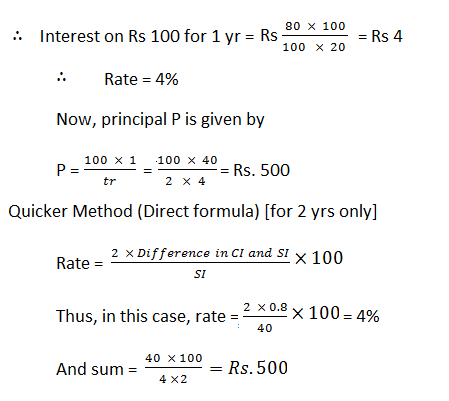New Student User - Use Code HELLO

# Simple and Compound Interest - Formulae, Concepts and Shortcuts

Today I am going to share various important questions that are repeatedly asked in bank exams. I have shared easiest way to solve these questions.### IMPORTANT FORMULAE

Let Principal = Rs. P, Time = t yrs and Rate = r % per annum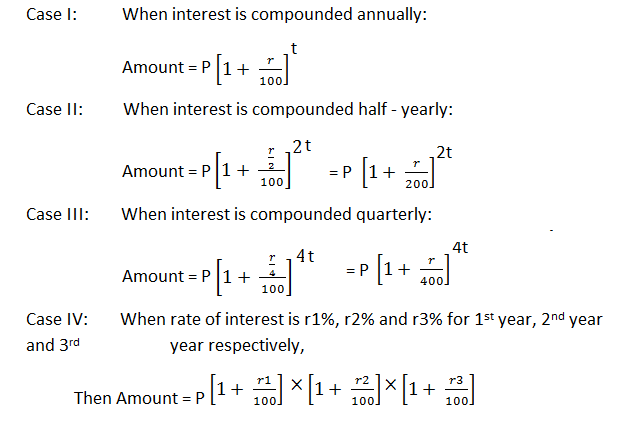#### Ques 1.

In what time will Rs 390625 amount to Rs 456976 at 4% compound interest?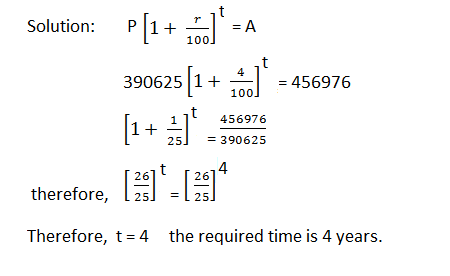#### Ques 2.

A sum of money placed at compound interest doubles itself in 4 yrs. In how many years will it amount to eight times itself ?
Solution :-

Quicker Approach:

X becomes 2x in 4 yrs.

2x becomes 4x in next 4 yrs.

4x becomes 8x in yet another 4 yrs.

Thus, x becomes 8x in 4 + 4 + 4 = 12 yrs.

#### Ques 3.

Find the least number of complete years in which a sum of money at 20% CI will be more than doubled.#### Ques 4.

A sum of money at compound interest amounts to thrice itself in three years. In how many years will it be 9 times itself?### Quicker Method: Remember the following conclusion:

If a sum becomes x times in y years at CI then it will be (x)n times in ny years.

Thus, if a sum becomes 3 times in 3 years it will be (3)2 times in 2 x 3 = 6 years.

Example:  If a sum deposited at compound interest becomes double in 4 years when will it be 4 times at the same rate of interest?

Solution: Using the above conclusion, we say that the sum will be (2)2 times in  2 x 4 = 8 years.

### TO FIND RATE

#### Ques 5.

At what rate per cent compound interest does a sum of money become nine-fold in 2 years?
Solution :-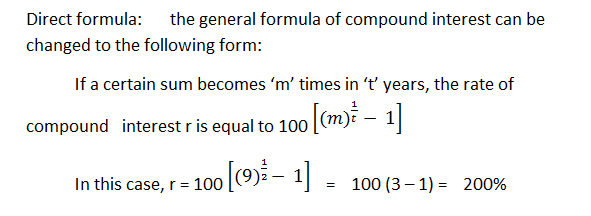#### Ques 6.

At what rate percentage (compound interest) will a sum of money become eight times in three years ?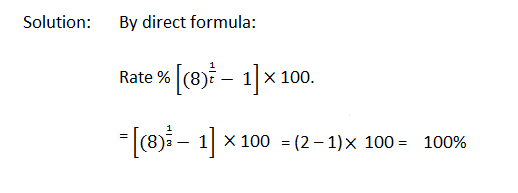#### Ques 7.

At what rate per cent compounded yearly will be Rs. 80,000 amount to Rs 88,200 in 2 years?

### GIVEN CI, To find SI and vice versa

#### Ques 8.

If the CI on a certain sum for 2 years at 3% be Rs. 101.50, what would be the SI?### GIVEN CI AND SI, TO FIND SUM AND RATE

#### Ques 9.

The compound interest on a certain sum for 2 yrs is Rs 40.80 and simple interest is Rs. 40.00. Find the rate of interest per annum and the sum.

Solution: A little reflection will show that the difference between the simple and compound interests for 2 yrs is the interest on the first year’s interest.

First year’s SI = Rs 40/2 = Rs 20

CI – SI = Rs 40.8 – Rs 40 = Rs 0.80

Interest on Rs 20 for 1 year = Re 0.80